TODO

# TODO: update screenshots and descriptions

## 2.1 Inserting math into the document

1. Place the cursor where you would like to insert the math.

2. Click Add-ons > hypatia > Insert/Edit Math.1. The hypatia math editor will open up. Use it to create the math you would like to insert. When you are done, click the Insert button.1. The math created in the editor should now be inserted into the document.## 2.2 A note about inline equation alignment:

Inline equations are not vertically aligned with the text in the same line. You will notice that they are positioned higher than text around.This is due to a restriction in Google Docs. If, in the future, Google changes this behavior, this alignment using hypatia will improve.

## 2.3 Editing math in the document

1. Select the math you would like to edit, then click Add-ons > hypatia > Insert/Edit Math.1. The hypatia math editor will open up. Use it to edit the math in the document. When you are done, click the Update button.1. The math you edited should now replace the selected math.# 3 Using the Sidebar

## 3.1 Opening the sidebar

1. Click Add-ons > hypatia > Show Sidebar.The sidebar contains several useful options and features in a place easily accessible to the user.

• Quick Start: A collection of tips for new users on how to quickly start using the editor.

• Actions: A list of actions you can perform. If the auto updates option is on, the list is updated automatically according to where the caret is placed in the document or what math is selected.

• Mini Editor: A tiny editor useful for editing short math. Using this editor is faster than opening the full editor but the mini editor is more limited in what you can do.

• Options: For customizing how the editor and sidebar behave.

• Editor window size: Use to set the size of the dialog box the editor opens in.

• Automatic sidebar updating: Turning this off will mean that the ‘Actions’ and ‘Mini editor’ won’t update automatically while you’re modifying your document. Instead you will need to move your cursor into the sidebar to get it to update.## 3.3 Inserting math with the mini editor:

1. Place the caret where you would like to insert a math (for centered math start a new paragraph).

1. Create the math you would like to insert in the mini editor. When you are done, click the Insert button.

1. The math created should now be inserted into the document.## 3.4 Editing math inside the mini editor:

1. Select the math you would like to edit. (Larger math may be opened in the full editor as usually more space is needed for its editing).

1. The mini editor should automatically load the selected math. Edit the math and when you are done, click the Update button.

1. The math you edited should now replace the selected math.## 3.5 Switching to the full editor:

1. Click Open in Full Editor to open the math currently in the mini editor in the full editor.1. The full hypatia editor should open up with the math from the mini editor math loaded.# 4 More About the Editor

The toolbar contains many of the supported math symbols organized into convenient submenus.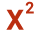Commonly used functions and symbols.E.g. α, β, sin, cos, sum, product, integral, derivative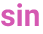Trigonometric functions, calculus, algebra.E.g. cos, sin, tan, log, ln, gcd, det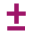Binary relations and operators.E.g. +,  =, ⊂, ≤,  ∈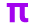Greek AlphabetE.g. α, β, γ, δ, ε, ζ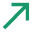Miscellaneous SymbolsE.g. →,⇒,∞,ℚ, ℝ,ℤ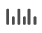UnitsE.g. cm, m, km, m2, m3, L, sec, g, kg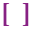BracketsE.g. (, |, {, [, <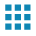Matrices and casesE.g. matrices with brackets/parentheses, determinants, tables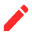AnnotationsE.g.comments, overtext, undertext

## 4.2 Using the toolbar to insert math symbols:

1. Place the caret where you would like to insert the math symbol.1. Click on the toolbar to open the submenu you want, then click on the symbol you would like to insert.1. The symbol you selected in the toolbar should now be inserted where the caret was.The toolbox will display the most recently used math symbols at the bottom of the editor.## 4.4 Opening the toolbox:

1. Click thebutton to open the Math Context Menu.

1. Then click the Show Toolbox checkbox.## 4.5 Using the toolbox:

1. Place the caret where you would like to insert a symbol.

1. Click on the symbol in the toolbox you would like to insert.1. The symbol you clicked in the toolbox should now be inserted where the caret was.## 4.6 Using the search menu:

1. Place the caret where you would like to insert a symbol.

1. Press the ‘\’ key on your keyboard to open the search menu.

1. Enter the name of the math symbol you would like to insert, then click on it.

1. Note: All math symbol can be accessed from the search menu (even those not available in the toolbar or toolbox).

1. The symbol you select in the search menu will be inserted where the caret was.## 4.7 Inserting matrices:

1. Place the caret where you would like to insert the matrix.

1. Click thetoolbar button, then click on the type of matrix you would like to insert.1. Enter the number of columns and rows you want the matrix to have.1. The matrix you specified should now be inserted where the caret was.

1. Note: The matrix will automatically come filled with zeros. You can use the TAB key to move along the positions in the matrix and insert entries.## 4.8 Modifying matrices:

1. Place the caret in the matrix you would like to modify.

1. Click thebutton to open the Modify Matrix Menu. From this menu, you can add/remove borders and add/remove rows and columns.

1. Click the option for the type of modification you would like to make.1. The matrix should now be modified according to the option you chose.## 4.9 Exchanging math symbols:

1. Place the caret in the math symbol you would like to exchange

1. Note: Not all symbols can be exchanged.

1. Click thebutton to open the Math Exchange Menu, then click on the math symbol you would like to exchange for.1. The math symbol should now be replaced by the symbol you chose in the math exchange menu.Types of single line math:

1. Inline math: Math on the same line as some text in a paragraph.1. Centered math: Math on a line by itself and centered.1. Multiline aligned equation: Multiple equations aligned along their equal signs.1. Multiline centered equation: Multiple lines of math with each line centered.## 4.11 Changing the math type:

1. Click thebutton to open the Math Context Menu.

1. Under Convert to you will see a list of math types you can convert the current math to. Click the option for the math type you would like to convert to.1. The math should now be converted to the type you choose in the math context menu.## 4.12 Table of Keyboard Shortcuts:

 Window/Linux Mac Copy Ctrl + c Cmd + c Cut Ctrl + x Cmd + x Paste Ctrl + v Cmd + v Select All Ctrl + a Cmd + a Undo Ctrl + z Cmd + z Redo Ctrl + y Cmd + Shift + z